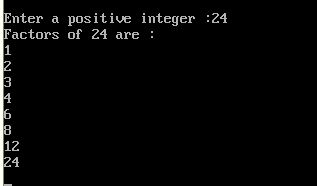UncleCoder.com

UncleCoder.com

Free programming examples and instructions

# C program to find the factors of a number

## C program for how to find factors of number

by Krishna

Posted on 27 Jul 2018 Category: C Views: 1838

C program to find the factors of a number

This program reads a  positive integer from the user and displays all the positive factors of that number. This program compiles successfully and output is also shown below.

``````#include <stdio.h>
#include <conio.h>
int main()
{
int num, i;

printf("Enter a positive integer: ");
scanf("%d",&num);

printf("Factors of %d are: \n", num);
for(i=1; i <= nuber; ++i)
{
if (num%i == 0)
{
printf("%d\n ",i);
}
}
getch();
return 0;
}
``````

OUTPUT